# Anti-eigenvalue

Jump to: navigation, search

The theory of anti-eigenvalues is a spectral theory based upon the turning angles of a matrix or operator. (See Eigen value for the spectral theory of stretchings, rather than turnings, of a matrix or operator.)

For a strongly accretive operator, i.e.,,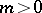, the first anti-eigenvalueis defined by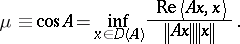(a1)

From (a1) one has immediately the notion of the angle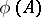: the largest angle through whichmay turn a vector. Any corresponding vectorwhich is turned by that angle is called a first anti-eigenvector. It turns out that, in general, the first anti-eigenvectors come in pairs. Two important early results were the minmax theorem and the Euler equation.

## Minmax theorem.

For any strongly accretive bounded operatoron a Hilbert space,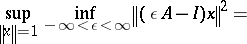(a2)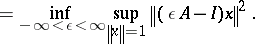Using the minmax theorem, the right-hand side of (a2) is seen to define(a3)

in such a way that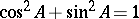. This implies an operator trigonometry (see [a1]).

## Euler equation.

For any strongly accretive bounded operatoron a Hilbert space, the Euler equation for the anti-eigenvalue functionalin (a1) is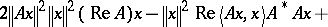(a4)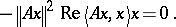Whenis a normal operator, (a4) is satisfied not only by the first anti-eigenvectors of, but by all eigenvectors of. Therefore the Euler equation may be viewed as a significant extension of the Rayleigh–Ritz theory for the variational characterization of eigenvalues of a self-adjoint or normal operator. The eigenvectors maximize the variational quotient (a1). The anti-eigenvectors minimize it. See [a2], [a3].

The theory of anti-eigenvalues has been applied recently (from 1990 onward) to gradient and iterative methods for the solution of linear systems; see [a5], [a6]. For example, the Kantorovich convergence rate for steepest descent,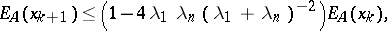where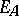denotes the-inner-product error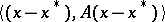, becomes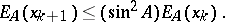Thus, the Kantorovich error rate is trigonometric. Similar trigonometric convergence bounds hold for conjugate gradient and related more sophisticated algorithms [a4]. Even the basic Richardson method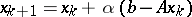(cf. also Richardson extrapolation) may be seen to have optimal convergence rate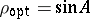. For further information, see [a5], [a6].